### Home > CALC > Chapter Ch10 > Lesson 10.4.1 > Problem10-142

10-142.
1. Calculate the following limits. Homework Help ✎

1.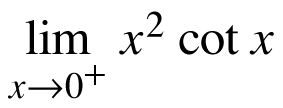2.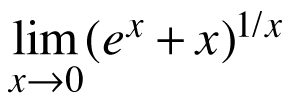Hint: Use natural log.

3.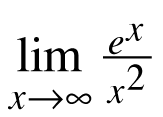4.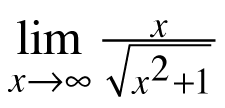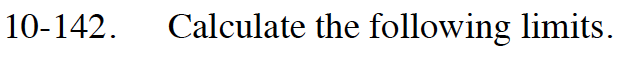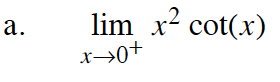Rewrite this limit as:

$\lim_{x\to 0^+}\frac{x^2}{\tan(x)}$

Then l'Hôpital's Rule can be used.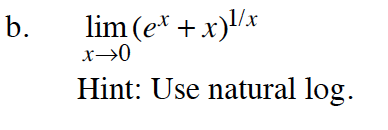$L=\lim_{x\to 0}(e^x+x)^{1/x}$

$\ln(L)=\ln\Big(\lim_{x\to 0}(e^x+x)^{1/x}\Big)$

$\ln(L)=\lim_{x\to 0}\frac{1}{x}\ln(e^x+x)=\lim_{x\to 0}\frac{\ln(e^x+x)}{x}$

Now l'Hôpital's Rule can be used.

When the limit is evaluated, the value is ln(L). Be sure to determine the value of L.